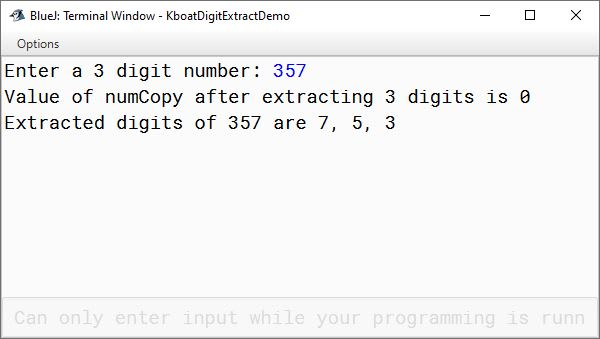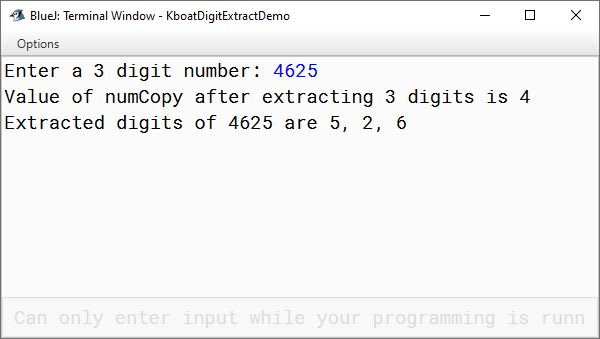# Solved Example: Extracting Digits of a Number

## ICSE Computer Applications

Now we will use the concepts of if-else statements we have learned so far and try to solve a few programming problems.

I want to start with explaining the method to extract the digits of a number. Every year in your board exam, one programming question comes where you need to extract the digits of the number to solve the problem. I will suggest you understand and practice it thoroughly.

Extracting digits of a number is very simple. When you divide a number by 10, the remainder is the digit in the unit’s place. You got your digit, now if you perform integer division on the number by 10, it will truncate the number by removing the digit you just extracted. You need to repeat these two steps till the number becomes zero to extract all digits of the number.

Let’s apply these steps on 357 and see if we can extract 3,5 and 7 from it.

Start by dividing 357 by 10. We get the remainder as 7. This gives us the first digit of the number. The quotient is 35. This quotient is the result of integer division of 357 by 10. So, our truncated number after extracting the first digit is 35.

Next, we repeat the steps with 35 as our new number. Dividing 35 with 10 gives 5 as the remainder. This gives us the second digit of the number. Our new truncated number is the quotient of this step i.e. 3.

We again repeat our steps with 3 as our new number. We get the third digit of the number as 3. The quotient is 0 which means that we have extracted all digits of the number.

So, by the end of this step we have all the 3 digits — 7, 5 & 3 of the number 357 with us. It is that simple to extract the digits of a number and secure one question of 15 marks in Section B of your board examination paper.

Now let’s translate these steps into Java code.

```import java.util.Scanner;

public class KboatDigitExtraction
{
public void extractDigits() {

Scanner in = new Scanner(System.in);
System.out.print("Enter a 3 digit number: ");
int number = in.nextInt();

int numCopy = number;

//Step 1
int digit1 = numCopy % 10;

//Step 2
numCopy /= 10;

//Step 3
int digit2 = numCopy % 10;

//Step 4
numCopy /= 10;

//Step 5
int digit3 = numCopy % 10;

//Step 6
numCopy /= 10;

System.out.println("Value of numCopy after extracting 3 digits is " + numCopy);

System.out.println("Extracted digits of "
+ number
+ " are "
+ digit1
+ ", "
+ digit2
+ ", "
+ digit3);
}
}```

I have coded all the steps of digit extraction that we discussed just now in the extractDigits method of KboatDigitExtraction class. Let’s go over the extractDigits method.

```Scanner in = new Scanner(System.in);
System.out.print("Enter a 3 digit number: ");
int number = in.nextInt();

int numCopy = number;```

We first ask the user to input a three-digit number. We take the input from the user with the help of Scanner class and store it in this `int` variable `number`. We then make a copy of `number` into `numCopy`. This step of making a copy of number is essential because we will truncate the number for extracting its digits and we don’t want to lose the original input given by the user.

```//Step 1
int digit1 = numCopy % 10;```

This is the first step of the process. Modulus operator returns the remainder of `numCopy` divided by 10. This gives us the first digit of the number which we store in `int` variable `digit1`.

```//Step 2
numCopy /= 10;```

In the second step we use the shorthand division operator to divide `numCopy` by 10 and store the result in `numCopy`. Notice both the variable `numCopy` and the literal 10 are of integer type so integer division is performed. This truncates the number by removing the digit in the unit’s place.

We repeat these steps to extract the second and third digit of the number.

```//Step 6
numCopy /= 10;

System.out.println("Value of numCopy after extracting 3 digits is " + numCopy);```

At step 6, value of `numCopy` should become zero for a 3-digit number. We will confirm this by looking at the output of this next println statement when we will execute the program.

```System.out.println("Extracted digits of "
+ number
+ " are "
+ digit1
+ ", "
+ digit2
+ ", "
+ digit3);```

After that, in the next println statement we print the three digits of the number that we extracted. Let’s execute the program and give 357 as the input.Notice, at step 6 after extracting 3 digits numCopy has become 0. This confirms that the input number had 3 digits.

I will execute the program again, this time giving a 4-digit number 4625 as input.Notice in the output that our program correctly extracts 3-digits of the number but value of numCopy is 4 and not 0 at step 6.

We can check the value of numCopy at the corresponding extraction step to validate that user is entering a value with the same number of digits we asked for. If we asked for a 3-digit number and user entered a 1-digit number, we can flag that as an invalid input.

We will validate user input for the correct number of digits in the next set of programs that we will write.